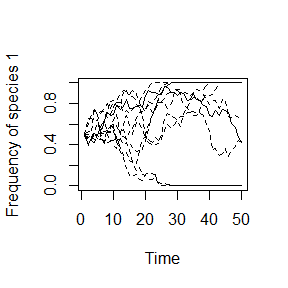## Online Box 5: R code to simulate drift, selection, and dispersal of two species in any number of patches: Figures 6.7-6.9 in the book

This code runs just one simulation

For Fig 6.7 (10-patch neutral model): J = 100, init.1 = 0.5*J, num.patch = 10, num.years = 50, fit.ratio.avg[] = 1, freq.dep[] = 0, m varies among panels

For Fig 6.8 (spatially variable selection - symmetrical): as in Fig. 6.7 except J = 1000, num.patch = 2, fit.ratio.avg[] <- c(1.2,(1/1.2))

For Fig 6.9 (spatially variable selection - asymmetrical): as in Fig. 6.8 except num.years = 100, fit.ratio.avg[] <- c(1.5,(1/1.1))

## specify parameters, initial conditions, and output matrix
num.years <- 50
num.patch <- 10
freq.1.mat <- matrix(nrow = num.years, ncol = num.patch)

J <- 100 # number of individuals PER PATCH
init.1 <- 0.5*J
COM <- matrix(nrow=J, ncol=num.patch)
COM[1:init.1,] <- 1; COM[(init.1+1):J,] <- 2

year <- 2

m <- 0
fit.ratio.avg <- vector(length=num.patch)
fit.ratio.avg[] <- 1
freq.dep <- vector(length=num.patch)
freq.dep[] <- 0

## record data (frequency of species 1) for year 1
freq.1.mat[1,] <- init.1/J

## run simulation
for (i in 1:(J*num.patch*(num.years-1))) {

## choose a patch where a death even will occur
patch <- sample(1:num.patch,1)

## calculate Pr.1 if dispersal occurs
if (runif(1) < m) {
Pr.1 <- sum(COM==1)/(J*num.patch)
} else {

## calculate Pr.1 if local reproduction (not dispersal)
freq.1 <- sum(COM[,patch]==1)/J; freq.2 <- 1 - freq.1
fit.ratio <- exp(freq.dep[patch]*(freq.1-0.5) + log(fit.ratio.avg[patch]))
Pr.1 <-  fit.ratio*freq.1/(fit.ratio*freq.1 + freq.2)
}

COM[ceiling(J*runif(1)),patch] <- sample(c(1,2), 1, prob=c(Pr.1,1-Pr.1))

## record data
if (i %% (J*num.patch) == 0) {
freq.1.mat[year,] <- colSums(COM==1)/J
year <- year + 1
}
}

## graph the results
plot(1:num.years, freq.1.mat[,1], type="l", xlab="Time",
ylab="Frequency of species 1", ylim=c(0,1))
for (i in 2:(num.patch)) {
lines(1:num.years,freq.1.mat[,i], type="l", lty=2, ylim=c(0,1))
}### Description of the code.

1. Unlike Boxes 1-4, we can now have more than two patches, with the number defined by num.patch. freq.1.mat is a matrix for output, as before, but with columns that are patches rather than separate simulations.
num.years <- 50
num.patch <- 10
freq.1.mat <- matrix(nrow = num.years, ncol = num.patch)
1. m is the dispersal parameter (m for migration, borrowed from the population genetics tradition). It defines the probability that the individual chosen for reproduction comes from the entire metacommunity rather than the local community.
m <- 0
1. With multiple patches, each can have its own value of the selection parameters, such that fit.ratio.avg and freq.dep are now vectors with num.patch elements. For example, fit.ratio.avg[] <- 1 gives all patches the same average fitness ratio (in this case 1). For two patches, fit.ratio.avg[] <- c(1.2,(1/1.2)) assigns the average fitness ratios of 1.2 and 1/1.2 to the two patches. For more patches, we add more values, separated by commas.
fit.ratio.avg <- vector(length=num.patch)
fit.ratio.avg[] <- 1
freq.dep <- vector(length=num.patch)
freq.dep[] <- 0
1. The metacommunity contains J*num.patch individuals, so each year must involve J*num.patch deaths. To choose a random individual for death, we first choose a patch by sampling an integer between 1 and num.patch at random.
for (i in 1:(J*num.patch*(num.years-1))) {

## choose a patch where a death even will occur
patch <- sample(1:num.patch,1)
1. Here is where dispersal happens. With probability m, we will define Pr.1 based only on the frequency of species 1 in the entire metacommunity, effectively making the reproducing individual a random selection from the metacommunity. The else statement says that we will otherwise (i.e., with probability 1 - m) choose the reproducing individual from the local community as in previous Boxes.
  if (runif(1) < m) {
Pr.1 <- sum(COM==1)/(J*num.patch)
} else { 
1. The graphs in Figures 6.7-6.9 have slightly different formatting. After calling the lines function, lty=2 makes all the lines (except the first) dashed, as in Figures 6.8 and 6.9. For all the lines to be solid (as in Figure 6.7), we can set lty=1 instead.
plot(1:num.years, freq.1.mat[,1], type="l", xlab="Time",
ylab="Frequency of species 1", ylim=c(0,1))
for (i in 2:(num.patch)) {
lines(1:num.years,freq.1.mat[,i], type="l", lty=2, ylim=c(0,1))